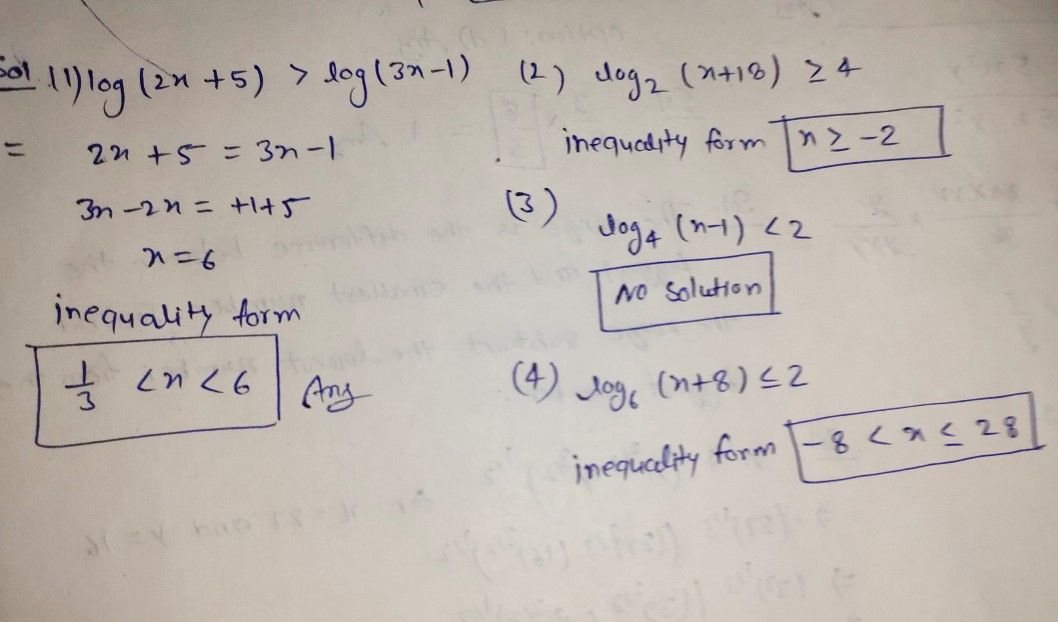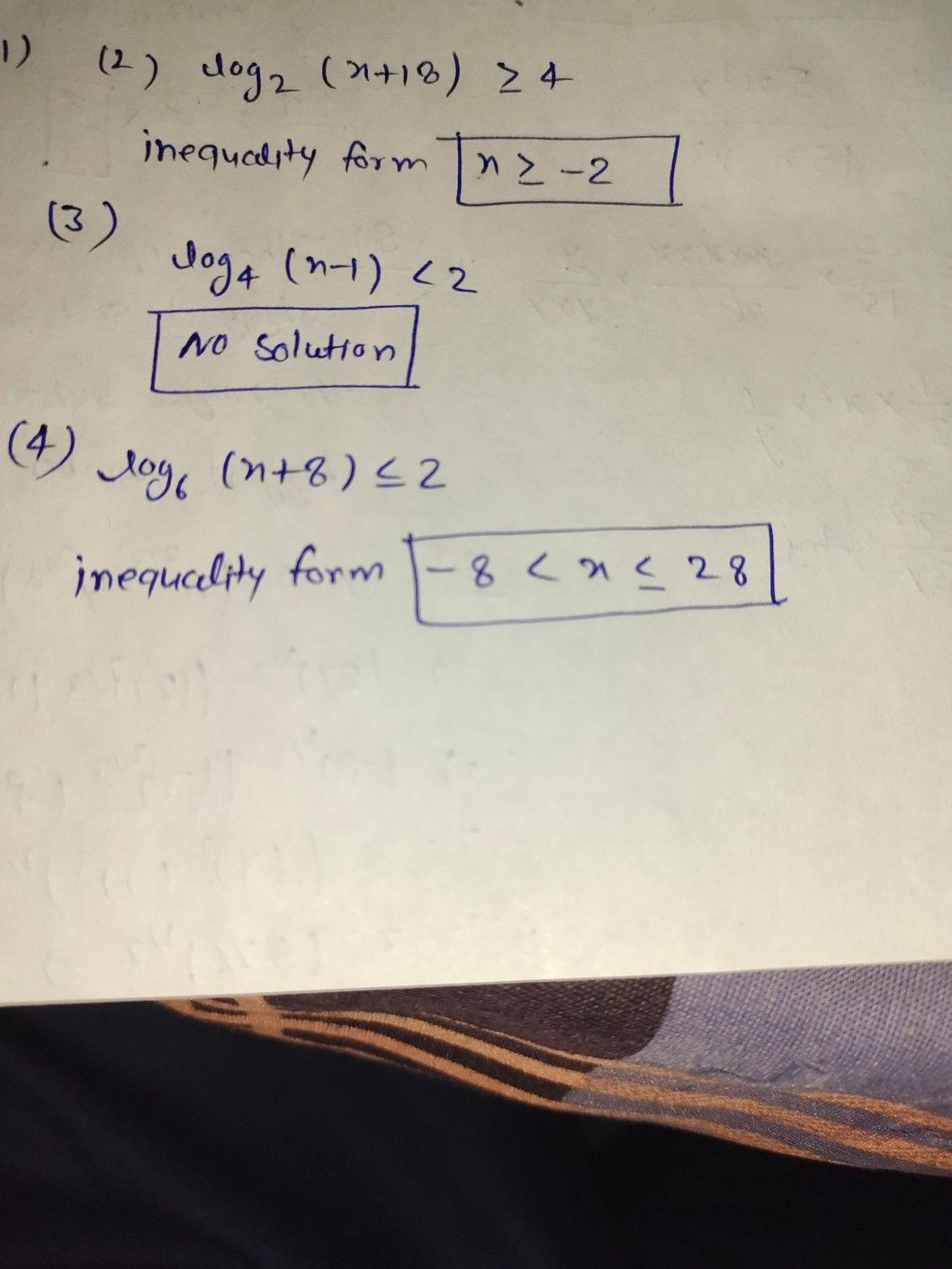Symbol
ProblemSolve the following logarithmic inequalities. $1$ $log\left(2x+5\right)>log\left(3x-1\right)$ $=$ $2$ $log2\left(x+18\right)\geq 4$ $3.$ $log4\left(x-1\right)<2$ $4$ $log _{6}\left(x+8\right)\leq 2$
10th-13th grade
Other
Search count: 111
SolutionQanda teacher - shraddha
Please wait 5 minStudent
ok po?Qanda teacher - shraddhaplz evaluate and and if u like my answer plz give additional reward coins as thankyou gift ☺️....and please give me 5 star.Student
where is the solution for 2, 3 and 4 maamQanda teacher - shraddhaStudent
i need step by step ma'am ?
if you will give me step by step solution i will rate you 5 stars and give you coins